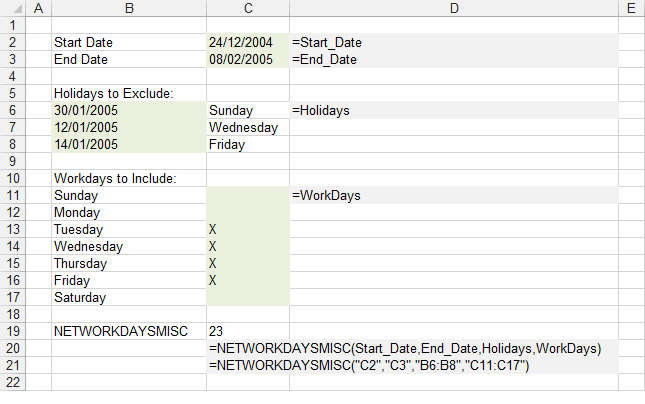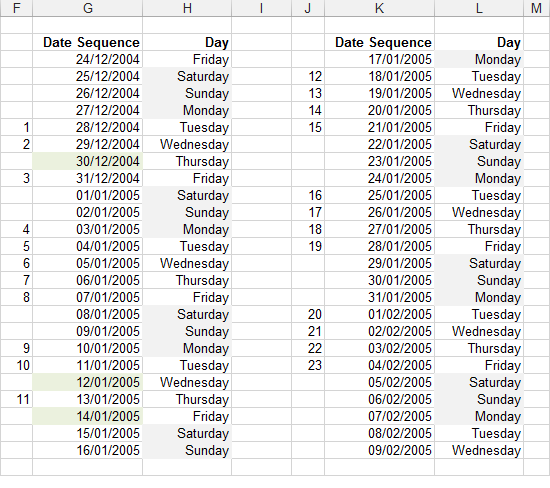# NETWORKDAYSMISC

Returns the number of days between two dates using a defined list of workdays.
For instructions on how to add a function to a workbook refer to the page under Inserting Functions

`Option Base 1    'lStartDate - The starting date'lEndDate - The finishing date'rgeHolidays - The dates to exclude from the working calendar, holidays and floating days.'rgeWorkdays - The days of the week that you want to include.Public Function NETWORKDAYSMISC(ByVal lStartDate As Long, _                                 ByVal lEndDate As Long, _                                 ByVal rgeHolidays As Range, _                                 ByVal rgeWorkDays As Range) As Long  Dim iweekdaycount As Integer Dim bhasvalidworkday As Boolean Dim bisvalidworkday As Boolean Dim lnewdate As Long Dim arholidays() As Long Dim iholidaycount As Integer Dim iarrayno As Integer Dim ldaycount As Long    If lStartDate = lEndDate Then NETWORKDAYSMISC = 0    If lStartDate = lEndDate Then Exit Function    bhasvalidworkday = False    For iweekdaycount = 1 To 7 Step 1       If rgeWorkDays.Item(iweekdaycount).Text <> "" Then          bhasvalidworkday = True          Exit For       End If    Next iweekdaycount    If bhasvalidworkday = False Then Call MsgBox("The rgeWorkDays parameter is incorrect")    If bhasvalidworkday = False Then Exit Function    ReDim arholidays(rgeHolidays.Count)    For iholidaycount = 1 To rgeHolidays.Count       If rgeHolidays.Item(iholidaycount).Value <> "" Then          arholidays(iholidaycount) = rgeHolidays.Item(iholidaycount).Value       Else          Exit For       End If    Next iholidaycount    ReDim Preserve arholidays(iholidaycount - 1)    lnewdate = lStartDate    ldaycount = 0    Do Until lnewdate = lEndDate       bisvalidworkday = True       If lStartDate < lEndDate Then lnewdate = lnewdate + 1       If lStartDate > lEndDate Then lnewdate = lnewdate - 1       If rgeWorkDays.Item(VBA.Weekday(lnewdate)).Text <> "" Then          For iarrayno = 1 To UBound(arholidays)             If lnewdate = arholidays(iarrayno) Then                 bisvalidworkday = False                 Exit For             End If          Next iarrayno          If bisvalidworkday = True Then             If (lStartDate - lEndDate) < 0 Then ldaycount = ldaycount + 1             If (lStartDate - lEndDate) > 0 Then ldaycount = ldaycount - 1          End If       End If    Loop    NETWORKDAYSMISC = ldaycount End Function `### Test